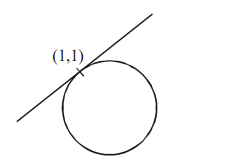# The line x=y touches a circle at the point (1,1).`
Question:

The line $x=y$ touches a circle at the point $(1,1)$. If the circle also passes through the point $(1,-3)$, then its radius is :

1. $3 \sqrt{2}$

2. 3

3. $2 \sqrt{2}$

4. 2

Correct Option: , 3

Solution:Equation of circle can be written as $(\mathrm{x}-1)^{2}+(\mathrm{y}-1)^{2}+\lambda(\mathrm{x}-\mathrm{y})=0$

It passes through $(1,-3)$

$16+\lambda(4)=0 \Rightarrow \lambda=-4$

So $(x-1)^{2}+(y-1)^{2}-4(x-y)=0$

$\Rightarrow x^{2}+y^{2}-6 x+2 y+2=0$

$\Rightarrow r=2 \sqrt{2}$